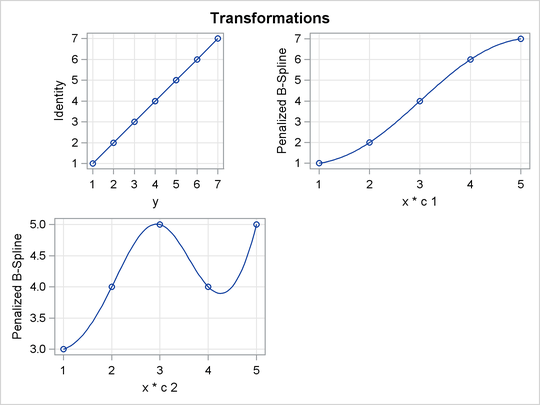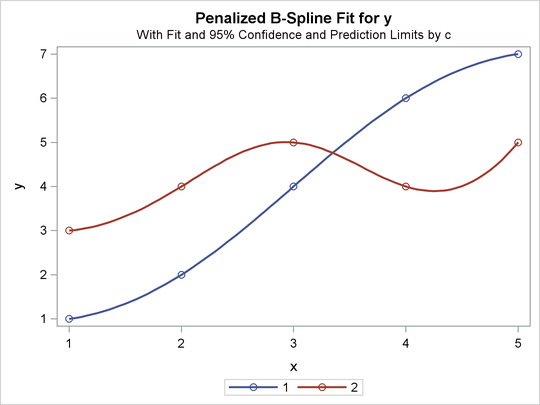### ODS Graphics

Statistical procedures use ODS Graphics to create graphs as part of their output. ODS Graphics is described in detail in Chapter 21: Statistical Graphics Using ODS.

Before you create graphs, ODS Graphics must be enabled (for example, by specifying the ODS GRAPHICS ON statement). For more information about enabling and disabling ODS Graphics, see the section Enabling and Disabling ODS Graphics in Chapter 21: Statistical Graphics Using ODS.

The overall appearance of graphs is controlled by ODS styles. Styles and other aspects of using ODS Graphics are discussed in the section A Primer on ODS Statistical Graphics in Chapter 21: Statistical Graphics Using ODS.

Some graphs are produced by default; other graphs are produced by using statements and options. You can reference every graph produced through ODS Graphics with a name. The names of the graphs that PROC TRANSREG generates are listed in Table 97.8, along with the required statements and options.

Table 97.8: Graphs Produced by PROC TRANSREG

ODS Graph Name

Plot Description

Statement & Option

BoxCoxFPlot

Box-Cox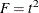MODEL & PROC, BOXCOX transform & PLOTS(UNPACK)

BoxCoxLogLikePlot

Box-Cox Log
Likelihood

MODEL & PROC, BOXCOX transform & PLOTS(UNPACK)

Box-Cox t or&
Log Likelihood

MODEL, BOXCOX transform

BoxCoxtPlot

Box-Cox t

MODEL & PROC, BOXCOX transform &
PLOTS(UNPACK)=BOXCOX(T)

Simple Regression and Separate Group Regressions

MODEL, a dependent variable that is not transformed, one non-CLASS independent variable, and at most one CLASS variable

Dependent Variable by
Predicted Values

MODEL, PLOTS=OBSERVEDBYPREDICTED

Penalized B-Spline
Criterion Plot

MODEL, PBSPLINE transform

Preference Mapping
Vector Plot

MODEL & PROC, IDENTITY transform & COORDINATES

Preference Mapping
Ideal Point Plot

MODEL & PROC, POINT expansion & COORDINATES

Residuals

PROC, PLOTS=RESIDUALS

RMSEPlot

Box-Cox Root Mean
Square Error

MODEL & PROC, BOXCOX transform &
PLOTS=BOXCOX(RMSE)

ScatterPlot

Scatter Plot of Observed Data

MODEL, one non-CLASS independent variable, and at most one CLASS variable, PLOTS=SCATTER

Variable Transformations

PROC, PLOTS=TRANSFORMATION

#### The PLOTS(INTERPOLATE) Option

This section illustrates one use of the PLOTS(INTERPOLATE) option for use with ODS Graphics. The data set has two groups of observations, `c` = 1 and `c` = 2. Each group is sparse, having only five observations, so the plots of the transformations and fit functions are not smooth. A second DATA step adds additional observations to the data set, over the range of `x`, with `y` missing. These observations do not contribute to the analysis, but they are used in computations of transformed and predicted values. The resulting plots are much smoother in the latter case than in the former. The other results of the analysis are the same. The following statements produce Figure 97.77 and Figure 97.78:

```title 'Smoother Interpolation with PLOTS(INTERPOLATE)';

data a;
input c y x;
output;
datalines;
1 1 1
1 2 2
1 4 3
1 6 4
1 7 5
2 3 1
2 4 2
2 5 3
2 4 4
2 5 5
;
```
```ods graphics on;

proc transreg data=a plots=(tran fit) ss2;
model ide(y) = pbs(x) * class(c / zero=none);
run;

data b;
set a end=eof;
output;
if eof then do;
y = .;
do x = 1 to 5 by 0.05;
c = 1; output;
c = 2; output;
end;
end;
run;

proc transreg data=b plots(interpolate)=(tran fit) ss2;
model ide(y) = pbs(x) * class(c / zero=none);
run;
```

The results with no interpolation are shown in Figure 97.77. The transformation and fit functions are not at all smooth. The results with interpolation are shown in Figure 97.78. The transformation and fit functions are smooth in Figure 97.78, because there are intermediate points to plot.

Figure 97.77: No Interpolation

 Smoother Interpolation with PLOTS(INTERPOLATE)

The TRANSREG Procedure

Univariate ANOVA Table, Penalized B-Spline Transformation
Source DF Sum of Squares Mean Square F Value Pr > F
Model 9 28.90000 3.211111 Infty <.0001
Error 12E-10 0.00000 0.000000
Corrected Total 9 28.90000

 Root MSE R-Square 0 1.0000 4.1 1.0000 0

Penalized B-Spline Transformation
Variable DF Coefficient Lambda AICC Label
Pbspline(xc1) 5.0000 1.000 2.642E-7 -66.4281 x * c 1
Pbspline(xc2) 5.0000 1.000 2.516E-7 -60.6430 x * c 2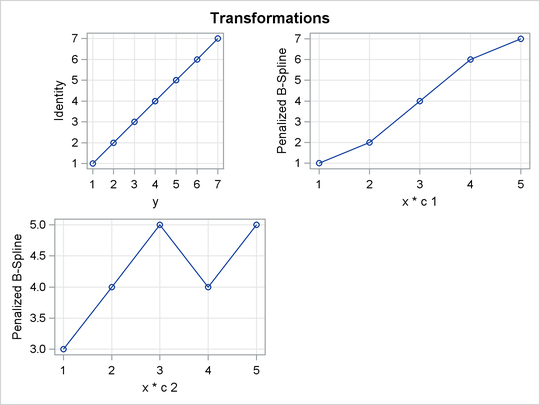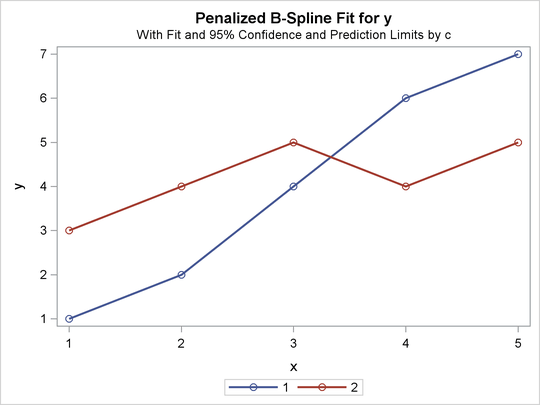Figure 97.78: Interpolation with PLOTS(INTERPOLATE)

 Smoother Interpolation with PLOTS(INTERPOLATE)

The TRANSREG Procedure

Univariate ANOVA Table, Penalized B-Spline Transformation
Source DF Sum of Squares Mean Square F Value Pr > F
Model 9 28.90000 3.211111 Infty <.0001
Error 12E-10 0.00000 0.000000
Corrected Total 9 28.90000

 Root MSE R-Square 0 1.0000 4.1 1.0000 0

Penalized B-Spline Transformation
Variable DF Coefficient Lambda AICC Label
Pbspline(xc1) 5.0000 1.000 2.642E-7 -66.4281 x * c 1
Pbspline(xc2) 5.0000 1.000 2.516E-7 -60.6430 x * c 2